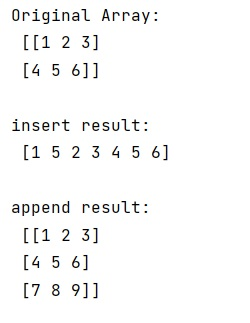# Difference between numpy.insert() and numpy.append() functions

Learn about the difference between numpy.insert() and numpy.append() functions in Python.
Submitted by Pranit Sharma, on March 01, 2023

NumPy is an abbreviated form of Numerical Python. It is used for different types of scientific operations in python. Numpy is a vast library in python which is used for almost every kind of scientific or mathematical operation. It is itself an array which is a collection of various methods and functions for processing the arrays.

## numpy.insert() Vs numpy.append() functions

In NumPy, we use the insert() function to insert values along the given axis before the given indices. It takes an input array, an object that defines the index or indices before which values are inserted, and the values which have to be inserted as the main 3 parameters. It also takes an optional parameter as an axis which represents the axis along which the values have to be inserted.

The only difference between numpy.insert() and numpy.append() is that numpy.append() is used to insert the values to the end of the array. It also takes an input array and the values that have to be inserted at the end of this array.

Let us understand with the help of an example,

## Python code to demonstrate the difference between numpy.insert() and numpy.append() functions

```# Import numpy
import numpy as np

# Creating a numpy array
arr = np.array([[1, 2, 3], [4, 5, 6]])

# Display original array
print("Original Array:\n",arr,"\n")

# Using insert
res = np.insert(arr,1, 5)

# Display result
print("insert result:\n",res,"\n")

# Using append
res = np.append(arr, [[7, 8, 9]], axis=0)

# Display result
print("append result:\n",res,"\n")
```

Output:Languages: » C » C++ » C++ STL » Java » Data Structure » C#.Net » Android » Kotlin » SQL
Web Technologies: » PHP » Python » JavaScript » CSS » Ajax » Node.js » Web programming/HTML
Solved programs: » C » C++ » DS » Java » C#
Aptitude que. & ans.: » C » C++ » Java » DBMS
Interview que. & ans.: » C » Embedded C » Java » SEO » HR
CS Subjects: » CS Basics » O.S. » Networks » DBMS » Embedded Systems » Cloud Computing
» Machine learning » CS Organizations » Linux » DOS
More: » Articles » Puzzles » News/Updates